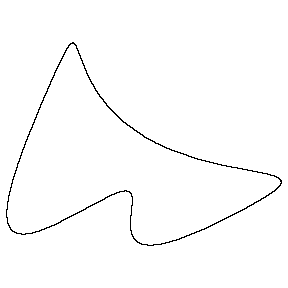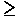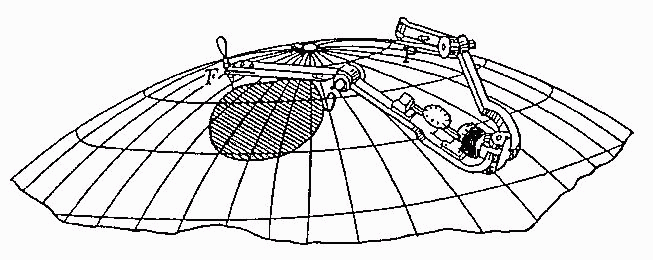Planimeters and Isoperimetric Inequalities

Why this page is viewed best with Internet Explorer or Sea MonkeyThe isoperimetric inequality for a region in the plane bounded by a simple closed curve states that L24pA, in which L is the length of the curve and A is the area of the region.  It's easily seen that equality holds when the curve is a circle.  In fact, equality holds only in this case.  There is a very simple and intuitive proof of the isoperimetric inequality based on how a planimeter works.
Proving the isoperimetric inequality requires tracing the boundary of the region with a planimeter in such a way that
• The tracer point goes around the boundary of the region as usual,
• The curve followed by the opposite end of the tracer arm encloses no area, and
• The planimeter makes a full rotation in the process.

This puts a limitation on the length of the planimeter that can be used.

Now consider the formula

AR - A =   l s  -  1/2 l l 2 Dq

from how a planimeter works. In this formula

• AR is the area enclosed by the tracer point; in this case it is the area A of the region,
• AL is the area enclosed by the opposite end of the tracer arm; as in the other applications, it is 0,
• l is the length of the tracer arm,
• s is the roll of the wheel,
• l is a parameter determined by the location of the wheel; in this case it is 1,
• Dq is the net rotation of the tracer arm; in this case it is 2p.

Plugging these values in we get

A  =   l s - p l 2.

A little algebra (completing the square in l) yields

s2 - 4pA = (A - pl 2)2/l 2 .

Now for a Key Observation:  The roll of the wheel, s, is less than or equal to the length of the boundary, L.  This is because the wheel is at the tracer point, and it records only the component of the motion of the tracer point that is perpendicular to the tracer arm. It follows that

 L2 - 4pA(A - pl 2)2/l 2.

This is stronger than the desired result! We leave it to the reader to ponder the geometric conditions under which equality occurs.

To see that the region has to be a circle when L2 = 4pA, consider the case when the length l of the tracer arm is the radius r of the circumscribing circle. We have

L2 - 4p(A - pr2)2/r2.

This is the same formula as above, of course, but it comes with an additional geometric interpretation. Suppose L2 = 4pA. It follows that A = pr2. Now the region is completely contained inside the circular disk. The only way for it to have the same area is for it to be the circular disk! This formula, along with this additional geometric interpretation, is known as a Bonnesen-type isoperimetric inequality.

A similar proof that works in spherical and hyperbolic geometry is contained in the paper Planimeters and Isoperimetric Inequalities on Constant Curvature Surfaces. In these spaces the isoperimetric inequality is

L24pA -  kA2,

where k is the curvature of the space:  k is 1/R2 for a sphere,  k  is negative for the hyperbolic plane.Spherical planimeter, Jacob Amsler, 1884

Two survey articles on isoperimetric inequalities by R. Osserman:
Bulletin of the American Mathematical Society, Nov. 1978
American Mathematics Monthly, Jan. 1979

Main planimeter page

Robert Foote
26 May 2009